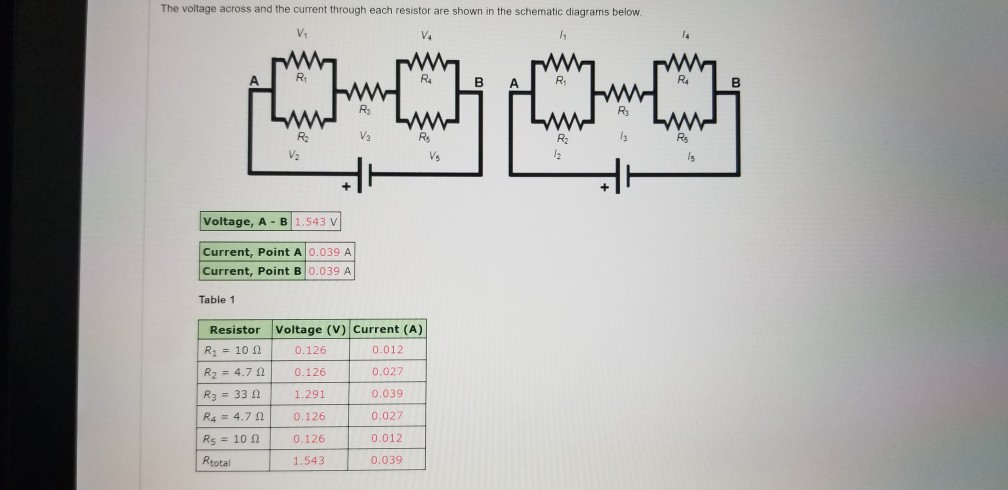# Problem: A. Using the schematic of the circuit, calculate the total resistance of the circuitB. Based on the theoretical value of R3 and the voltage measured at R3, calculate the theoretical value of the current I3 using Ohm’s LawC. What do the results suggest about the current coming into a junction and the current leaving a junction

###### FREE Expert Solution

Equivalent resistance for 2 resistors in parallel:

$\overline{){{\mathbf{R}}}_{{\mathbf{eq}}}{\mathbf{=}}\frac{{\mathbf{R}}_{\mathbf{1}}{\mathbf{R}}_{\mathbf{2}}}{{\mathbf{R}}_{\mathbf{1}}\mathbf{+}{\mathbf{R}}_{\mathbf{2}}}}$

95% (218 ratings)###### Problem DetailsA. Using the schematic of the circuit, calculate the total resistance of the circuit

B. Based on the theoretical value of R3 and the voltage measured at R3, calculate the theoretical value of the current I3 using Ohm’s Law

C. What do the results suggest about the current coming into a junction and the current leaving a junction